# Errata: Contour integral in derivation of Bode's integral formula is analyzed incorrectly

Location: page 340, line 3-9

In the computation of the integral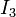, the analysis of the value of the contour integral is incorrect. The corrected text is shown below in blue:

Next we consider the integral. For this purpose we split the contour into three parts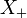,and, as indicated in Figure 11.16. We can then write the integral asThe contouris a small circle with radiusaround the pole. The magnitude of the integrand is of the order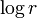, and the length of the path is. The integral thus goes to zero as the radiusgoes to zero. Since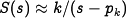close to the pole, the argument ofdecreases by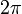as the contour encircles the pole. On the contoursandwe therefore haveHenceand we get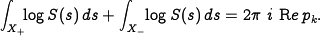Repeating the argument for all polesin the right half plane, letting the small circles go to zero and the large circle go to infinity givesSince complex poles appear as complex conjugate pairs,, which gives Bode's formula (11.19).

(Contributed by D. MacMynowski, A. Asimakapoulos, 22 Nov 2010)